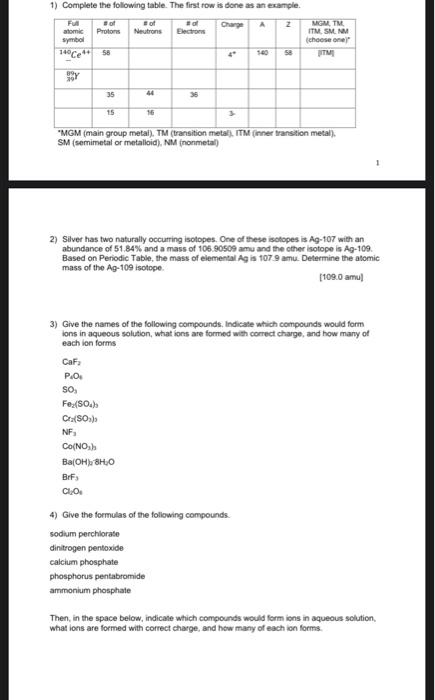Home / Expert Answers / Chemistry / 1-complete-the-following-table-the-first-row-is-done-as-an-example-34-mgm-main-group-metai-tm-pa772

# (Solved): 1) Complete the following table. The first row is done as an example. "MGM (main group metai). TM ( ...1) Complete the following table. The first row is done as an example. "MGM (main group metai). TM (transition metal). ITM (inner transition metal). SM (semimetal or metalloid), NM (nonmetal) 2) Silver has two naturally occurring isotopes. One of these isolopes is $$\mathrm{Ag}-107$$ with an abundance of $$51.84 \%$$ and a mass of $$106.90509$$ amu and the cther isotope is $$\mathrm{Ag}-109$$. Based on Periodic Table, the mass of elemental Ag is $$107.9$$ amu. Determine the atomic mass of the Ag-109 isotope. [109.0 amu] 3) Give the names of the following compounds. Indicate which compounds would form ions in aqueous solution, what ions are formed with correct charge, and how many of each ion forms $$\mathrm{CaF}_{2}$$ $$\mathrm{POO}_{4}$$ $$\mathrm{Fe}_{2}\left(\mathrm{SO}_{4}\right)_{3}$$ $$\mathrm{Cr}_{2}\left(\mathrm{SO}_{3}\right)_{3}$$ $$\mathrm{NF}_{3}$$ $$\mathrm{Co}\left(\mathrm{NO}_{3}\right)_{3}$$ $$\mathrm{Ba}_{\left(\mathrm{OH}_{3}\right.} \mathrm{BH}_{4} \mathrm{O}$$ $$\mathrm{Br}_{3} \mathrm{Cl}_{4}$$ 4) Give the formulas of the following compounds. sodium perchlorase dinitrogen pentoxide calcium phosphate phosphorus pentabromide ammonium phosphate Then, in the space below, indicate which campounds would form ions in aqueous solution, what ions are formed with correct charge, and hew many of each ion forms.

We have an Answer from Expert